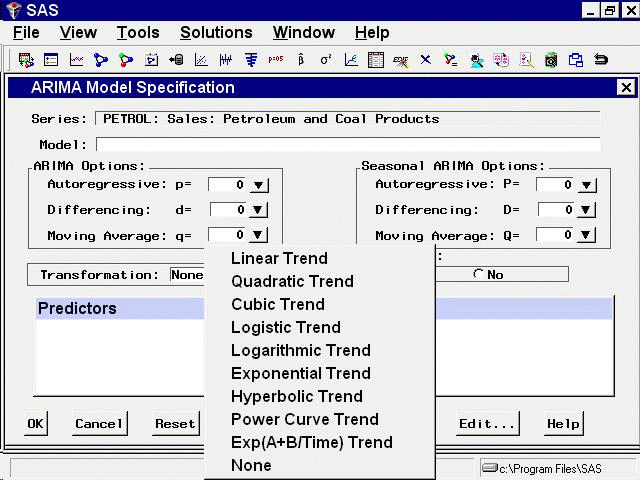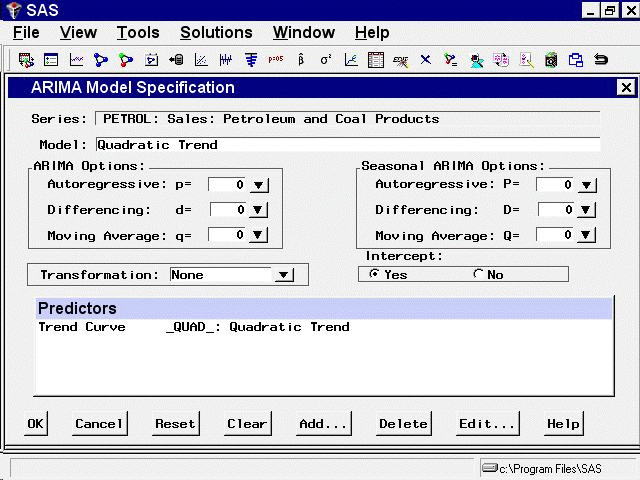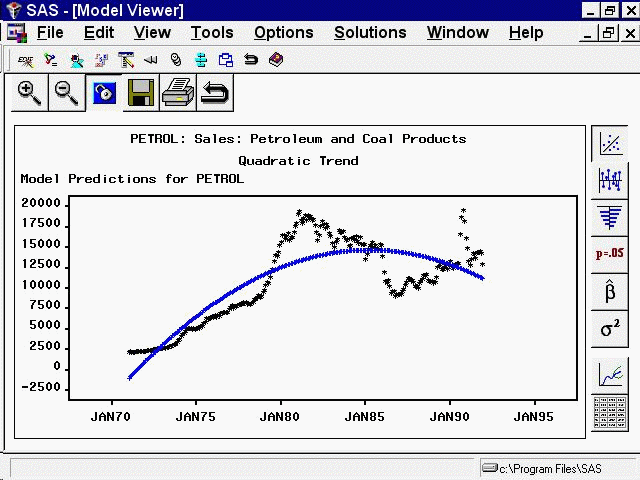# Using Predictor Variables

## Time Trend Curves

From the Develop Models window, select `Fit ARIMA Model`. From the ARIMA Model Specification window, select `Add` and then select `Trend Curve` from the menu (shown in Figure 50.1). A menu of different kinds of trend curves is displayed, as shown in Figure 50.5.

Figure 50.5: Time Trend Curves MenuThese trend curves work in a similar way as the Linear Trend option (which is a special case of a trend curve and one of the choices on the menu), but with the Trend Curve menu you have a choice of various nonlinear time trends.

Select `Quadratic Trend`. This adds a quadratic time trend to the Predictors list, as shown in Figure 50.6.

Figure 50.6: Quadratic Trend SpecifiedNow select the `OK` button. The quadratic trend model is fit and added to the list of models in the Develop Models window. The Model Viewer displays a plot of the quadratic trend model, as shown in Figure 50.7.

Figure 50.7: Quadratic Trend ModelThis curve does not fit the PETROL series very well, but the View Model plot illustrates how time trend models work. You might want to experiment with different trend models to see what the different trend curves look like.

Some of the trend curves require transforming the dependent series. When you specify one of these curves, a notice is displayed reminding you that a transformation is needed, and the Transformation field is automatically filled in. Therefore, you cannot control the Transformation specification when some kinds of trend curves are specified.

See the section Time Trend Curves in Chapter 53: Forecasting Process Details, for more information about the different trend curves.